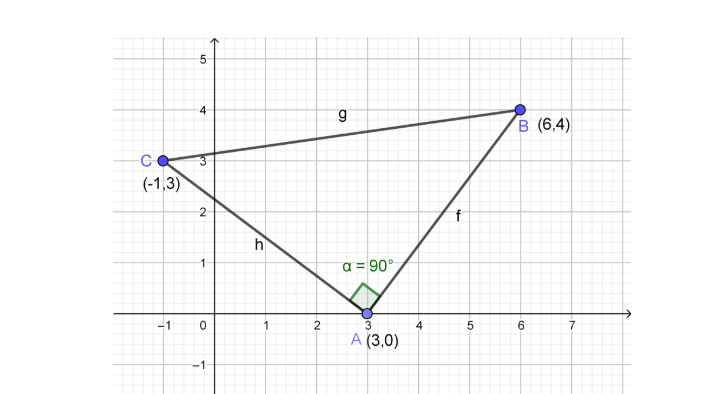Courses
Courses for Kids
Free study material
Offline Centres
MoreLast updated date: 07th Dec 2023
Total views: 384.6k
Views today: 8.84k

# The points (3,0) (6,4) and (-1,3) are vertices of a right angled triangle. Show that it is an Isosceles triangle.Verified
384.6k+ views
Hint: To prove the given statement use the distance formula and prove that any two sides of the triangle are equal, which is the necessary and sufficient condition for an isosceles triangle.

To prove this we should draw a right angled triangle with given points as,
A (3,0), B (6,4) and C (-1,3)Now to prove any triangle as an isosceles triangle we should know the definition of it i. e. “A triangle having its two sides equal in length is called an Isosceles Triangle.”
Therefore we will measure all the three lengths of the right angled triangle and we will see that any of its two sides are equal or not,
To find the lengths of sides of a triangle from its vertices we should know the distance formula given below,
Formula:
If P $({{x}_{1}},{{y}_{1}})$and Q $({{x}_{2}},{{y}_{2}})$then distance between PQ is given by,
PQ$=\sqrt{{{\left( {{x}_{2}}-{{x}_{1}} \right)}^{2}}+{{\left( {{y}_{2}}-{{y}_{1}} \right)}^{2}}}$
By using the formula we will find the distances as follows,
1) Length of AB,
As we know A (3,0) and B (6,4)
Assume A $({{x}_{1}},{{y}_{1}})$ and B $({{x}_{2}},{{y}_{2}})$
Therefore we can write,
${{x}_{1}}=3,{{y}_{1}}=0$
${{x}_{2}}=6,{{y}_{2}}=4$
Now from distance formula we can write the length AB as,

AB$=\sqrt{{{\left( {{x}_{2}}-{{x}_{1}} \right)}^{2}}+{{\left( {{y}_{2}}-{{y}_{1}} \right)}^{2}}}$
$\therefore AB=\sqrt{{{\left( 6-3 \right)}^{2}}+{{\left( 4-0 \right)}^{2}}}$
$\therefore AB=\sqrt{{{\left( 3 \right)}^{2}}+{{\left( 4 \right)}^{2}}}$
$\therefore AB=\sqrt{9+16}$
$\therefore AB=\sqrt{25}$
$\therefore AB=5$…………………………………………………. (1)
2) Length of BC,
As we know B (6,4) and C (-1,3)
Assume B $({{x}_{1}},{{y}_{1}})$ and C $({{x}_{2}},{{y}_{2}})$
Therefore we can write,
${{x}_{1}}=6,{{y}_{1}}=4$
${{x}_{2}}=-1,{{y}_{2}}=3$
Now from distance formula we can write the length AB as,

BC$=\sqrt{{{\left( {{x}_{2}}-{{x}_{1}} \right)}^{2}}+{{\left( {{y}_{2}}-{{y}_{1}} \right)}^{2}}}$
$\therefore BC=\sqrt{{{\left( -1-6 \right)}^{2}}+{{\left( 3-4 \right)}^{2}}}$
$\therefore BC=\sqrt{{{\left( -7 \right)}^{2}}+{{\left( -1 \right)}^{2}}}$
$\therefore BC=\sqrt{49+1}$
$\therefore BC=\sqrt{50}$
$\therefore BC=\sqrt{25\times 2}$
$\therefore BC=5\sqrt{2}$ ………………………………………………… (2)
3) Length of AC,
As we know B (6,4) and C (-1,3)
Assume B $({{x}_{1}},{{y}_{1}})$ and C $({{x}_{2}},{{y}_{2}})$
Therefore we can write,
${{x}_{1}}=3,{{y}_{1}}=0$
${{x}_{2}}=-1,{{y}_{2}}=3$
Now from distance formula we can write the length AB as,

BC$=\sqrt{{{\left( {{x}_{2}}-{{x}_{1}} \right)}^{2}}+{{\left( {{y}_{2}}-{{y}_{1}} \right)}^{2}}}$
$\therefore AC=\sqrt{{{\left( -1-3 \right)}^{2}}+{{\left( 3-0 \right)}^{2}}}$
$\therefore AC=\sqrt{{{\left( -4 \right)}^{2}}+{{\left( 3 \right)}^{2}}}$
$\therefore AC=\sqrt{16+9}$
$\therefore AC=\sqrt{25}$
$\therefore AC=5$……………………………………………….. (3)

From equation (1) and (3) we can say that,
Length of AB = Length of AC
Therefore we can say that,
In a right angled triangle given by the vertices (3,0) (6,4) and (-1,3) have two sides equal, and hence the right angled triangle is an Isosceles triangle.

Note: While using the distance formula, give notations to the points as shown below, so that there will be no confusion while substituting the value in formula, to use the distance formula AB$=\sqrt{{{\left( {{x}_{2}}-{{x}_{1}} \right)}^{2}}+{{\left( {{y}_{2}}-{{y}_{1}} \right)}^{2}}}$ use the notations for A and B as,
A $({{x}_{1}},{{y}_{1}})$ and B $({{x}_{2}},{{y}_{2}})$ for A (3,0) and B (6,4) therefore you will get the values easily and can easily put in formula without confusion.